Get instant live expert help with Excel or Google Sheets“My Excelchat expert helped me in less than 20 minutes, saving me what would have been 5 hours of work!”

#### Post your problem and you'll get expert help in seconds

Your message must be at least 40 characters
Our professional experts are available now. Your privacy is guaranteed.

# Learn How to Calculate Overtime in Excel

The total worked hours and overtime hours calculations are dependent on employees’ start time and finish time. So, we can easily keep track of these times to calculate overtime by comparing the total hours worked with the regular hour threshold. This article will step through the process of calculating overtime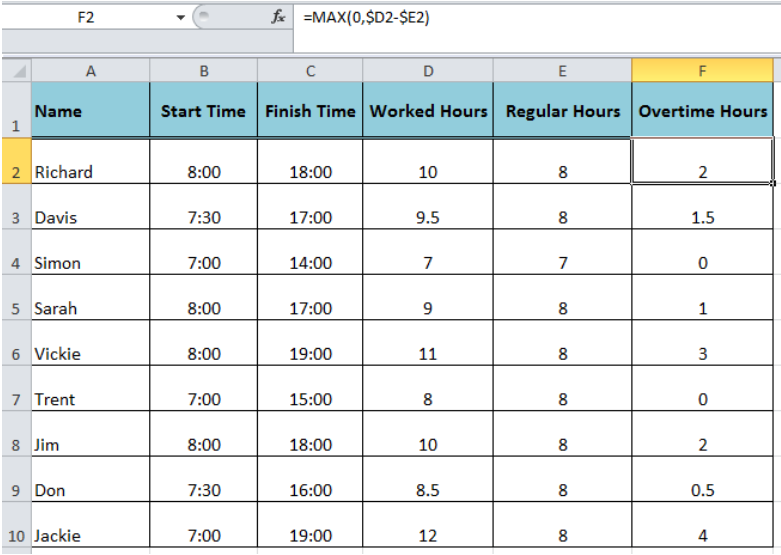Figure 1. Overtime Calculation in Excel

First of all, we need to set up our spreadsheet to track the start time and finish time of employees’ shift, such as column B and C. This time tracking is important to make calculations for worked hours, regular hours and overtime hours, such as column D, E and F respectively in our example.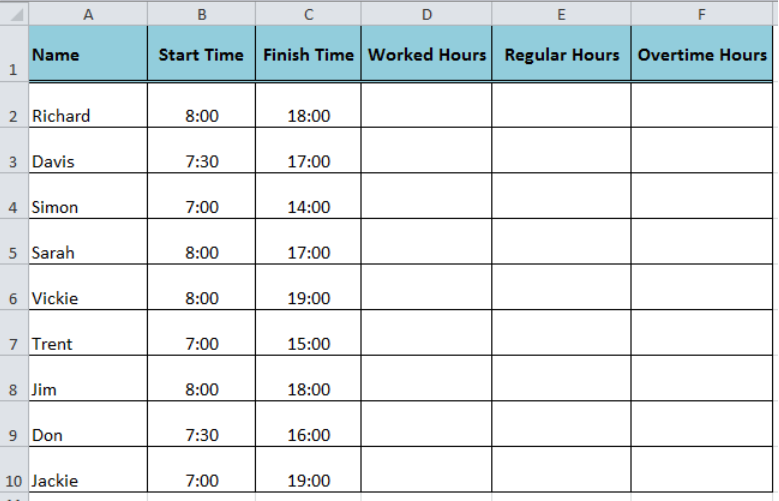Figure 2. Setting Up Spreadsheet

## Calculating Worked Hours

First, we need to calculate the total worked hours based on employees’ shift start and finish timings. This is done by simply subtracting start time from the finish time. As Excel natively handles the time as a fraction of the day, therefore we need to convert this fraction to decimal hours to make worked hours calculations simple and easy by multiplying the difference of start and finish timings by 24, such as;

`=(Finish_time - Start_time)*24`

And if there is a case, where shift crosses the midnight then we also need to calculate elapsed time in total worked hours calculation. In this case, we need to make some adjustments in basic above worked hours calculation formula, such as;

`=(Finish_time - Start_time+(Finish_time < Start_time))*24`

So, the final formula to calculate “Worked Hours” in this example would be as follows as entered in cell D2;

`=(C2-B2+(C2<B2))*24`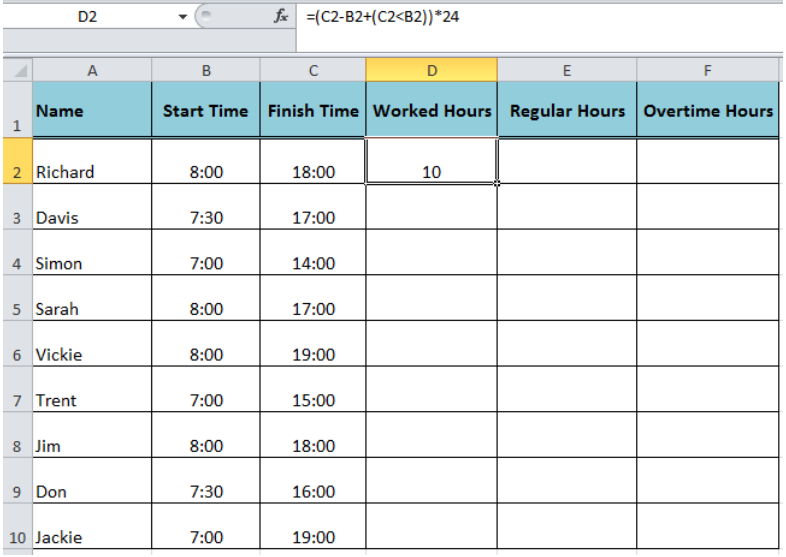Figure 3. The formula to Calculate Worked Hours

## Calculating Regular Hours

The regular hours’ calculation is essential to calculate overtime in Excel and it is done by comparing defined regular hours, say 8 hours, with worked hours calculated in column D, whichever is smaller. For this, we use the MIN function in column E to calculate “Regular Hours” by entering the following formula in cell E2;

`=MIN(8,D2)`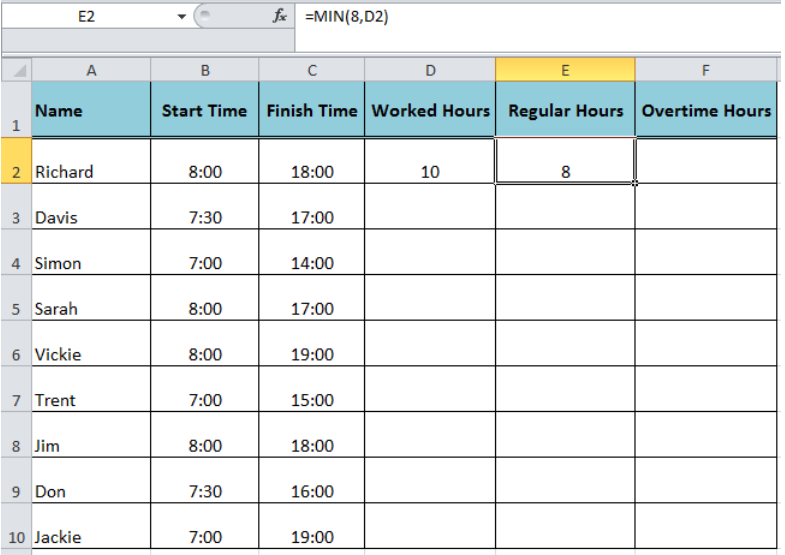Figure 4. Calculating Regular Hours

## Calculating Overtime Hours

After calculating worked hours and regular hours, now we are able to calculate overtime in Excel. This is simply worked hours minus regular hours or applying the MAX formula in cell F2, such as;

`=MAX(0,\$D2-\$E2)`Figure 5. Calculating Overtime Hours

Now select the cells D2, E2, and F2 and drag down this selection to apply this set of formulas to other rows in the spreadsheet as shown below;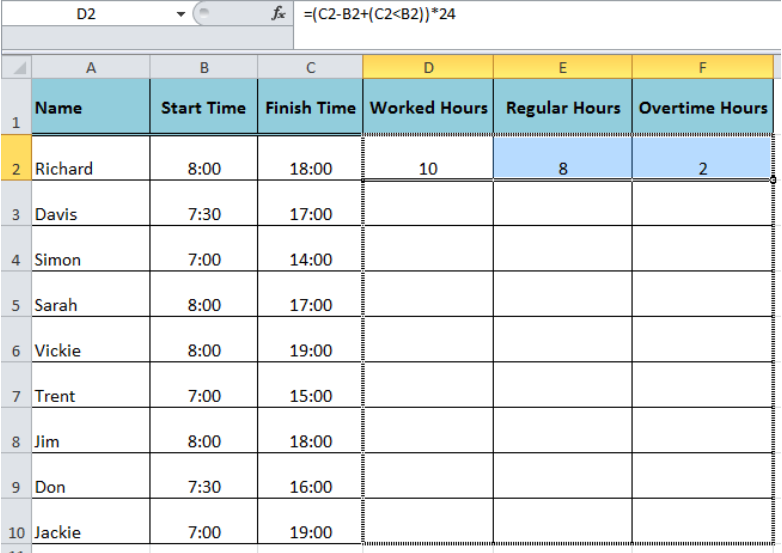Figure 6. Dragging the Formulas Set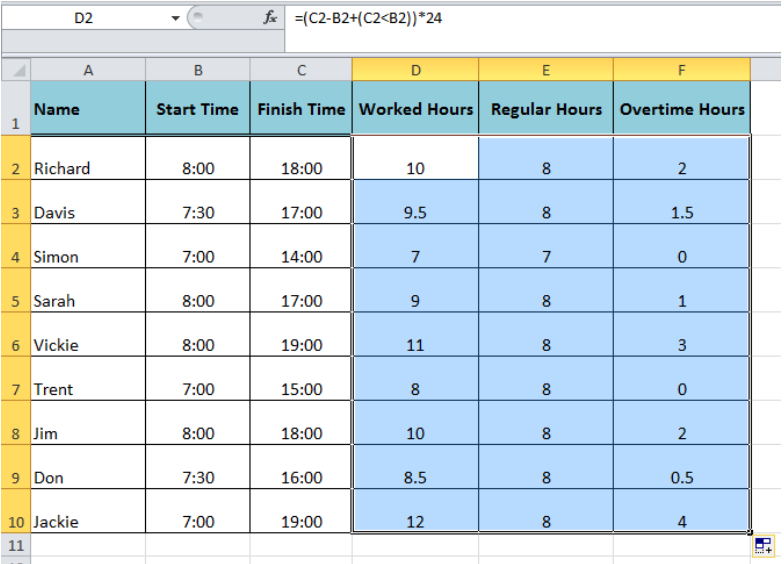Figure 7. Final Preview of Calculations

## Worked Hours Calculation with Break Times in Shift

If we incorporate break timings in our spreadsheet, such as “Lunch Start” and “Lunch End” timings, then we need to adjust the worked hours formula to subtract these break hours from worked hours in order to correctly calculate overtime in Excel. So, the formula for worked hours would be as follows:

`=((E2-B2+(E2<B2))-(D2-C2))*24`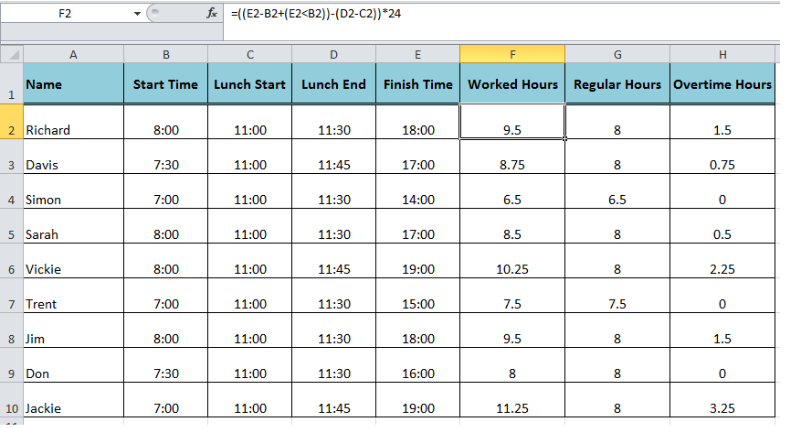Figure 8. Calculating Worked Hours by Subtracting Break Hours

## Instant Connection to an Expert through our Excelchat Service

Most of the time, the problem you will need to solve will be more complex than a simple application of a formula or function. If you want to save hours of research and frustration, try our live Excelchat service! Our Excel Experts are available 24/7 to answer any Excel question you may have. We guarantee a connection within 30 seconds and a customized solution within 20 minutes.